## Greedy Algorithm

An algorithm used to recursively construct a Set of objects from the smallest possible constituent parts.

Given a Set ofIntegers (,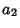, ...,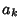) with, a greedy algorithm can be used to find a Vector of coefficients (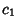,, ...,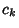) such that(1)

whereis the Dot Product, for some given Integer. This can be accomplished by lettingfor, ...,and setting(2)

Now define the difference between the representation andas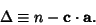(3)

Ifat any step, a representation has been found. Otherwise, decrement the Nonzeroterm with least, set all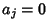for, and build up the remaining terms from(4)

for, ..., 1 untilor all possibilities have been exhausted.

For example, McNugget Numbers are numbers which are representable using only. Takingand applying the algorithm iteratively gives the sequence (0, 0, 3), (0, 2, 2), (2, 1, 2), (3, 0, 2), (1, 4, 1), at which point. 62 is therefore a McNugget Number with(5)

If any Integercan be represented withor 1 using a sequence (,, ...), then this sequence is called a Complete Sequence.

A greedy algorithm can also be used to break down arbitrary fractions into Unit Fractions in a finite number of steps. For a Fraction, find the least Integersuch that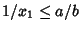, i.e.,(6)

whereis the Ceiling Function. Then find the least Integersuch that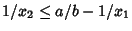. Iterate until there is no remainder. The Algorithm gives two or fewer terms forand, three or fewer terms for, and four or fewer for.

Paul Erdös and E. G. Strays have conjectured that the Diophantine Equation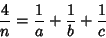(7)

always can be solved, and W. Sierpinski conjectured that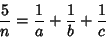(8)

can be solved.

See also Complete Sequence, Integer Relation, Levine-O'Sullivan Greedy Algorithm, McNugget Number, Reverse Greedy Algorithm, Square Number, Sylvester's Sequence, Unit Fraction